topology

# the 3-star

The 3-star is basically the intersection of 3 perpendicular planes.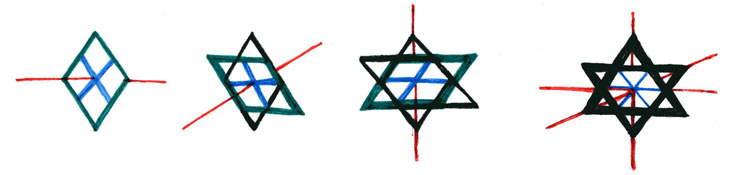Begin by taking one plane, adding another at right angles, and then a third at right angles to both.

Here is a moving .gif to demonstrate the process.The 3-star has many practical purposes for measuring not only 3-space shapes, but also as a basis for plotting the coordinate systems of 4-manifolds.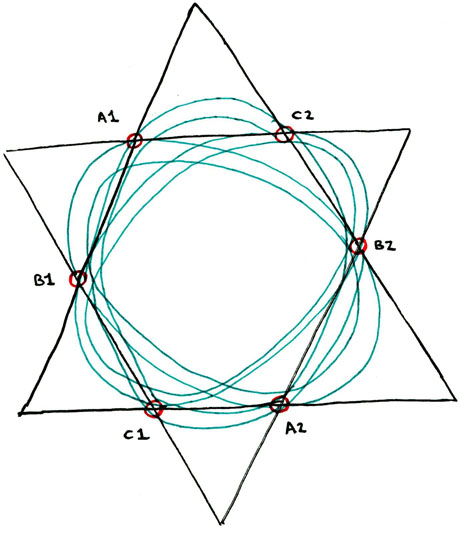Here, we see the 3-star used to mount 3 perpendicular toroids, forming a gyroscope. The points of intersection of each toroid with the other three are marked A1, A2, B1, B2, C1 and C2.

It should be briefly noted that the toroid CA intersects the toroid AB at A1 and A2, the toroid AB intersects the toroid BC at B1 and B2, and the toroid BC intersects the toroid CA at C1 and C2.

It may be deduced then, that the covering set for each of the intersecting plane spaces can be folded around so that A1 connects to A2, B1 to B2, and C1 to C2. Doing so renders the three perpendicular toroids as shown, such that they form a gyroscope whose operation describes a hypersphere.

Here is a more detailed examination of the three toroids.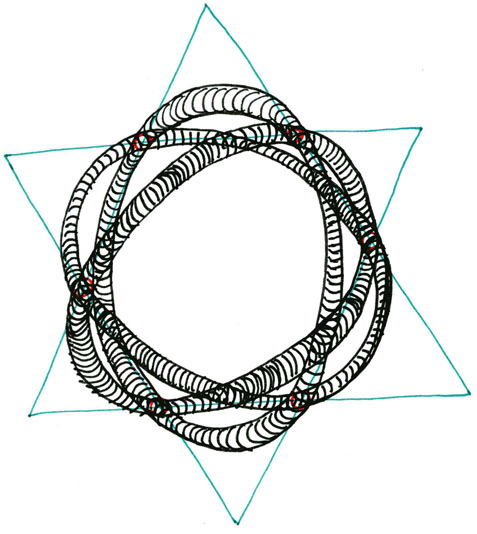# Modeling the fourth dimension with light and motion

We can depict and thereby easily understand the three standard accepted dimensions comprising the spatial component of our continuum. We model this as the intersection at right angles of three planes, and depict it using diagrams such as the 3-star described above.

However representing the fourth dimension, whether we understand it spatially or temporally, or spatially and temporally, is not quite as easy.

For example, it is possible to understand four spatial shapes by depicting the possible shadows that they cast into the third dimension, and then modeling these, usually as flat diagrams drawn in the second spatial dimension. However, these 3-space shadows of 4-space objects, no matter how thoroughly we can model them in either stasis or motion, are limited as 2-spatial diagrams, as well as being limited to representing the 4-space shapes only at certain angles. For example, a cube seen from above a corner casts a hexagonal shadow on a sheet of paper, and so, too, is the 3-space tesseract the shadow of only one possible position of the, otherwise much more difficult to visualise, 4-cube.

Another way of modeling the fourth dimension is by examining 3-spatial shapes over time. Let us understand the temporal dimension as beiing the measure, perpendicular to the three intersecting planes of 3-space, of a 3-spatial object from itself. For example, imagine a cube sitting on my desk. The difference between this cube and itself can be rendered as the passage of time. Of course, in this model, we cannot see any immediate difference between one state of the cube and another from one moment to the next. So, if we want to model dynamic change over duration we must work with moving parts. Consider, instead of the cube, a gyroscope sitting on my desk. Now, as the gyroscope is set in motion and spins, there is an immediately visualisable difference between itself in one state and itself in another from one moment to the next. Hence, we can use motion as a measurement of the temporal direction, which, again, operates at a fourth right angle to the standard intersection of planes in 3-space.

Now, ideally, to consider the full scope of the fourth dimension, we should combine the shadow effect of one dimension being visualisably diagramable on the next dimension beneath it, AND the measurement of motion as being perpendicular to the 3-spatial matter upon which it acts. So, for example, let us consider the following two diagrams.The first measures several different directions of motion on the model of the gyroscope. Here we see that the three planes intersecting at right angles may be depicted as the axes of three great arcs. Each great arc has its own orientation of motion, and the momentum caused by the motions of the combined three great arcs causes further dynamic rotation to occur. In the case of this diagram, that motion is depicted by the insertion of a 2-space axis at 45° between the perpendicularly oriented axes of the three great arcs. As the great arcs revolve around the origin point of these conjoined axes, their motion creates the momentum that drives the rotation of the 2-space axis. The 3-arcs revolve and the 2-axis rotates. To add even one more step of motion to the model, I have pinioned the entire gyroscopic contraption upon a stable pivot, such that the 3-arcs revolve, causing the 2-axis to rotate, causing the whole gyroscope to revolve around the pivot point. (for reference, the revolution of the great arcs is depicted by the three colour arrows, the rotation of the 2-axis by the solid black semi-circular arrows, and the revolution of the contraption around the pivot by the black outlined white arrows.) This diagram maps the confluence of nine directions of motion, and if the pivot point had been rendered such that, rather than being stable, it followed a precessional concourse (imagine the pivot-beam tracing out a circle around the base of its stable stand), then the diagram would model motion in at least ten different directions. Of course, it is equally easy to continue adding motion in as many different directions as possible, however for the purpose of this exposition we needn't worry about the implications of doing so.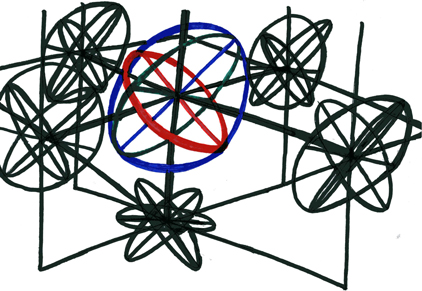The second diagram depicts the same essential model, that being the gyroscope, as well as the shadows that it casts in five of the six cardinal directions. It should become evident upon consideration of this diagram that only three of these shadows are actually necessary for a thorough examination of light's effects on the model, the other three shadows merely being equal and opposite mirror images of the first three. For the sake of brevity the motions of these shadows are not depicted, however it is hoped that the viewer of this diagram should be able to piece these together for themselves.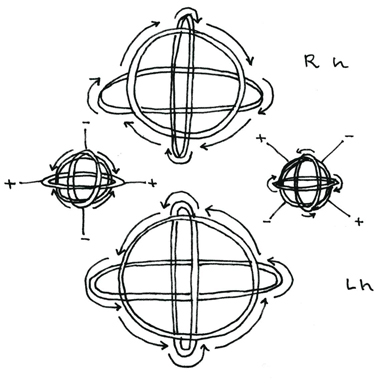There are four basic stations of motion for the tripartite gyrsoscope. These represent different spin states for the 3 great arcs of the gyroscope. The right hand (Rh) and left hand (Lh) spin states are simple spinors with only one direction for all three great arcs. These create simple vortecis in a fluid medium, where the rotation of the spin of the gyroscope drives the liquid substance into a simple vortex in the center of the gyroscope. The other stabe positions of the gyroscope involve either one or two of the great arcs moving in an opposite direction from the other(s). These, depicted on the left and right of the diagram, orient themselves with positive and negative positions where the fluid medium disrupted by the motion of the gyroscope will be drawn toward the positive positions and away from the negative positions. In the model on the left, the positive poles are positioned relative to the static horizontal great arc, while in the model on the right, the positive poles are positioned relative to the static position of the greater vertical arc. Because there are dual polar positions for the other models, we can call them scalar rather than spinor vector motions.

----------------------------------------------------------------------------------------------------------------

# some topology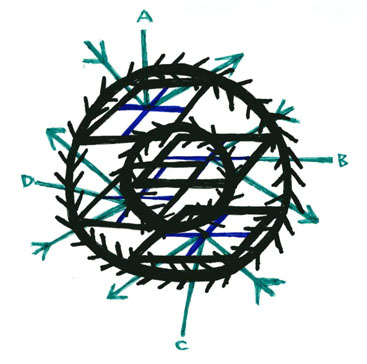In this figure we see 4 coordinate system plane spaces tangent to a toridal 4-manifold. Intersecting the origin points of the four coordinate system plane spaces are four vertices. These four vertices represent the magnitude (inward arrows) and the direction (outward arrows) of four resultant vectors (labeled A, B, C, and D). While the depicted coordinate system plane spaces are all parallel, three of the vectors (A, C and D) occur at right angles to them, while one of the vectors (B) occurs parellel to its vertex's plane space coordinate system. These four angles define the 4-dimensionality of the toroid manifold. The 4-dimensionality of the toroid manifold is further depicted by its motion: the interior circle operates clockwise, while the exterior circle operates counterclockwise. This form of motion is callled "counter-rotation." The motion on the surface of the torus "involutes" from around the outside toward the hole at the center. The involution of the toroid's surface causes the magnitude of the inward vectors to reflect off the toroid surface along an outward direction. The resultants of the direction/magnitude of the vectors are all perpendicular to the toroid surface, and since they all operate at right angles to one another as well as to the surface of the torus, the four resultant vectors measure the 4-dimensionality of the torus manifold.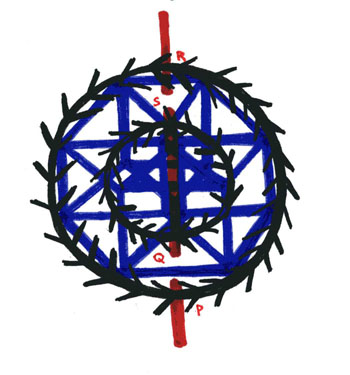In this figure we see the four coordinate system plane spaces conjoined as an interior and exterior face of two cubes conjoined in a 4-manifold tesseract. An axis has been added that penetrates the torus between points P and Q and points S and R.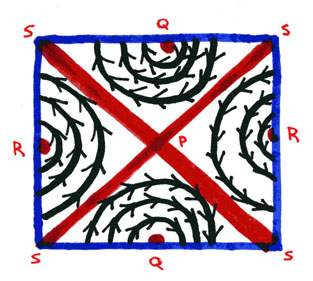In this figure we see the phase portrait of the torus mapped onto a single plane space. The points P and Q and points S and R are the same as in the last diagram.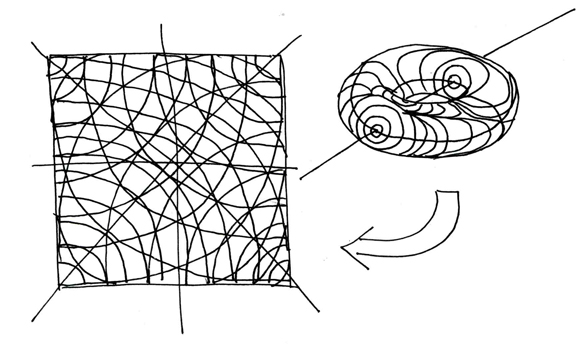Here is the standard measure of how a torus is mapped onto a flat, two-dimensional plane. The complete plane is called the "plane space." There are two ways to express vibrations of a plane space. These are topologically and using matrix mathematics. The two are really the same, since the "neighborhood set" "spans the space" only when its "vector matrix" is "orthonormal."

----------------------------------------------------------------------------------------------------------------

this information is all © 2004 - Jonathan Barlow Gee